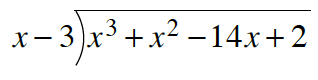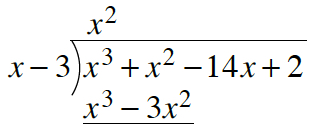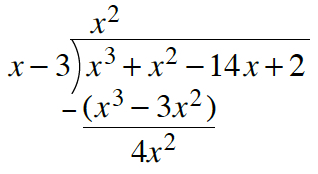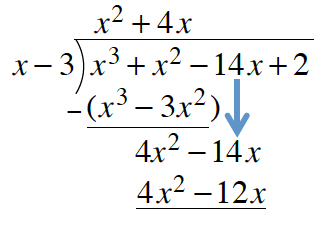### Home > PC > Chapter 7 > Lesson 7.2.3 > Problem7-63

7-63.

Complete the following division problem. Express any remainder as a fraction.

$(x^3+x^2-14x+2)\div$$(x-3)$

Use an area model as shown in the steps for problem 3-54, or use long division.

Long Division:

Problem written as long division, side labeled, x minus 3, inside labeled, x cubed, + x squared, minus 14 x, + 2.

Added, above x cubed, is x squared, below x cubed is x cubed, below x squared, is minus 3 x squared.

Added: parentheses around x cubed minus 3 x squared, with negative sign in front of left parenthesis, with underscore below. Below underscore, is 4 x squared.

Added: down arrow below minus 14 x, and minus 14 x is added next to the 4 x squared. Below and aligned, is 4 x squared, minus 12 x.Continue this process.